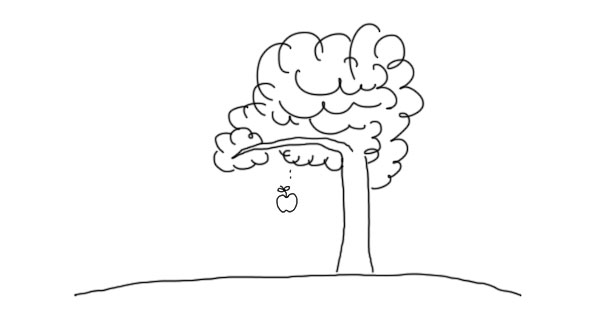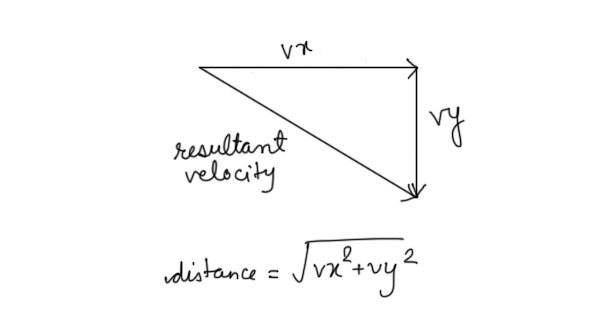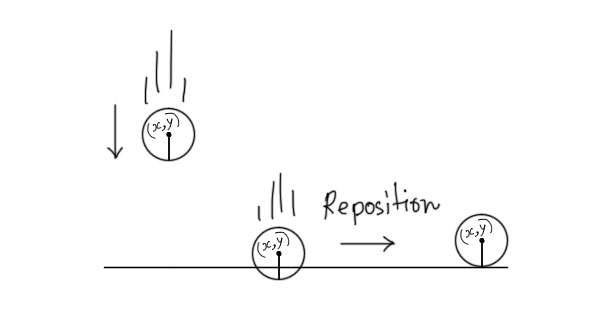# Basics of Implementing Gravity with HTML5 CanvasWhen creating some canvas experiments like a particle emission system or a game, gravity can be a key feature to implement. Figuring out how to implement gravity on an object in terms of coding can be a bit confusing. We’ll try to comprehend it, by putting it into code in a simplified manner. I liek simplicity!

What's the one thing every developer wants? More screens! Enhance your coding experience with an external monitor to increase screen real estate.

## Understanding Gravity

You probably already know what gravity is. Pick an object from the ground, lift it up to some extent and then release it from hands. What will happen ? It’ll fall down, right. That’s because Earth attracts any object with a force. This force is called gravity. It affects the acceleration of an object that in turn affects the velocity. Velocity is speed with direction.

When an object falls, it’s direction is downwards. This means that working with gravity is as simple as manipulating the acceleration of an object in the Y axis.

## Getting to Code

First we’ll code a little canvas experiment where a ball moves upwards and then downwards. Take a look at this demo. What are we doing ? Basically, we draw a ball at the bottom of the canvas’s 2D context. Drawing a ball is easy, just need to use the `arc` method.

```var canvas = document.querySelector('canvas');
var ctx = canvas.getContext('2d');
var W = canvas.width = window.innerWidth;
var H = canvas.height = window.innerHeight;

// Velocity x
var vx = 0;
// Velocity y
var vy = (Math.random() * -10) - 5;
```

Simple and basic stuff, right ?

```function Ball() {
this.x = canvas.width / 2;
this.y = canvas.height - this.radius - 20;

this.draw = function(ctx) {
ctx.fillStyle = 'black';
ctx.beginPath();

ctx.arc(
this.x,
this.y,
0,
Math.PI*2,
false
);

ctx.closePath();
ctx.fill();
}
}

var ball = new Ball();
```

A Simple `Ball` Constructor with properties for radius, x position, y position and drawing the ball. Finally, we do the animation:

```(function renderFrame() {
requestAnimationFrame(renderFrame);
ctx.clearRect(0, 0, W, H);

ball.x += vx;
ball.y += vy;

ball.draw(ctx);
}());
```

The `renderFrame` method moves the ball upwards which is quite easy. All we need to do is add some constant velocity on the x and y axis. For the x axis, we just add 0. Actually, we could have even omitted the `ball.x += vx;` for now. As for the y axis, we generate a random number using `Math.random()` (as you saw before) and multiply the number with -10. We push it further by deducting -5. Now we have a negative number that we can add on `ball.y` to apply negative velocity, i.e, move it in the upward direction.

It is very important to understand that `vx` and `vy` are just constant values that are added on the x and y positions of the ball, that’s it. When adding a value on the x and y position of the ball and redrawing it, the ball appears to be in a different position in the next frame. This way the ball moves at a velocity of `Math.sqrt(vx*vx + vy*vy)` pixels per frame. We just applied Pythagorean theorem.You can relate this entire demo to a kid who stands in a playground, holding a ball in his hands. Suddenly, for some reason, he’s induced with too much happiness and throws the ball upwards in the sky. Now he’s no superman, which means the ball will not go out of earth and enter the space. Right? The ball must come down, due to gravity.

## Gravity as Acceleration

So, how do we make the ball in our experiment obey the laws of gravity ?

First, we’ll need to define our gravity value.

```var gravity = 0.5; // Just a random value
```

Next, it is important to understand that gravity is acceleration that affects velocity. Gravity attracts an object downwards. This means, gravity will affect `vy` only. So all we need to do is, add our gravity to `vy`. This piece of code in our `renderFrame` method will do the job:

```vy += gravity; // adding gravity to velocity on y axis
```

Yes! We just added gravity to our experiment producing some realistic results.

## Repositioning Object

Hey! Wouldn’t it be nice if we reposition the object as soon as it exits the canvas from bottom to prevent the animation from coming to a halt ?

The idea behind repositioning is that, as soon as an object moves off the canvas, you place it at a new position (repositioning). The new position in our case can be the one that we used in our `Ball` Constructor.

```if (
ball.x + ball.radius > canvas.width ||
ball.x - ball.radius < 0 ||
ball.y + ball.radius > canvas.height// ||
) {

// Re-positioning on the base
ball.x = canvas.width / 2;

// If we do not re-set the velocities
// then the ball will stick to bottom

// Velocity x
vx = 0;
// Velocity y
vy = (Math.random() * -15) - 5;
}
```We’re checking whether the ball is off the canvas or not. That’s what the if conditions check for. Note, adding and subtracting radius is important. In our case we don’t really need to check whether the ball goes out of x axis or not nor do we need to check whether it goes into the negative y axis space or not (as it is going to come down eventually anyway). But usually, you’ll need to check for all 4 cases.

## Did We Just Make a Bouncing Ball ?

Kind of, yes. If you want a proper realistic bouncing ball then we’ll need to introduce a bounce factor and multiply the negative of that to `vy` instead of setting a new `vy` everytime.

Defining a bounce factor:

```var bounce_factor = 0.8;
```

Applying it to the velocity in the `renderFrame` method:

```// Velocity y
// vy = (Math.random() * -15) - 5;
vy *= -bounce_factor;
```

But, what is a Bounce Factor ? Bounce Factor is the force with which the ball should rebound when it hits a surface. A value of 1 denotes a bounce factor of 100% which means the ball is going to bounce back with cent percent force that it hit the surface with. This means that a bounce factor of 1 will make the ball reach the original position on rebound if there is NO change in the x position. Similarly, a bounce factor of 0.3 would mean rebounding with 30% force. In our case we used 0.8 (80%).

If you are still a bit confused as to why a bounce factor of 1 will make the ball travel same distance after rebound as it travelled before rebound, then you can try some random values with some of the motion equations:

• s = ut + 1/2*at^2
• v = u + at

With the first equation, find the initial distance (before rebound). Then use the second equation to find the final velocity. Finally, use the final velocity as initial velocity in the first equation to find the final distance (after rebound). Don’t worry, nothing too complex. Basic physics and math.

It is important to note that we have to use a negative bounce factor during multiplication. This will negate the velocity causing the ball to move in the upward direction.

## Conclusion

Isn’t this really interesting ? From trying to understand the basics of gravity with a simple experiment, we just made few alterations to our code and ended up building a realistic bouncing ball. We even learnt the concept of repositioning objects which you’ll probably use in many of your canvas experiments.

Here’s a codecast by Kushagra that he made few weeks ago on the same concept. It’s a bit buggy (doesn’t stops bouncing/vibrating), but still does some good job as far as teaching is concerned.

Recommended from our users: Dynamic Network Monitoring from WhatsUp Gold from IPSwitch. Free Download## Author: Rishabh

Rishabh is a full stack web and mobile developer from India. Follow me on Twitter.

## 3 thoughts on “Basics of Implementing Gravity with HTML5 Canvas”

1.Alex says:

Great job!
I ask you to change the code by inserting, instead of a path …. an IMAGE. Maybe a basketball (a jpg or png!) that bouncing instead of the black ball. It would be more and more realistic…

Regards,
Alex

1.Caitlin says:

I’m with Alex. How can you do this with an image?

1.Aidan Beggs says:

Although this is a little late, you could simply draw the image at the X and Y coordinates, instead of drawing a circle(I.E. ctx.drawImage(img, ball.x, ball.y) instead of ctx.fillStyle = ‘black’;
ctx.beginPath();

ctx.arc(
this.x,
this.y,